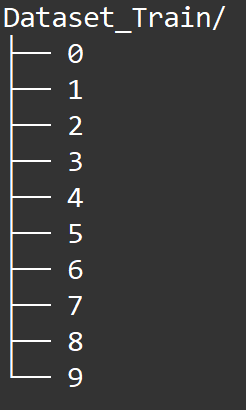## 張量 — Tensor

TensorFlow 的計算單位，稱之為張量(Tensor)。而計算過程就是流(Flow)

• 通常定義張量的物理學或傳統數學方法，是把張量看成一個多維數組，當變換座標或變換基底時，其分量會按照一定變換的規則，這些規則有兩種：即協變或逆變轉換。
• 通常現代數學中的方法，是把張量定義成某個向量空間或其對偶空間上的多重線性映射，這向量空間在需要引入基底之前不固定任何座標系統。

N等於一的時候，就是向量；N等於二，就是矩陣。意即「向量」和「矩陣」的稱呼，只是張量的特例。

## 流 — Flow

TensorFlow 的流，需要把所有的節點設置好了以後，用「sess.run()」來啟動計算圖。

# include<stdio.h>
# include<stdlib.h>

int main()
{
int A = 10;
int B = 20;
int C = A + B;
printf("%d\n", C);
system("pause");
}

import tensorflow as tf

# 宣告常數
A = tf.constant(50)
B = tf.constant(100)

# 運算子
C = A + B

# 啟動計算圖
with tf.Session() as sess:
print(sess.run(C))# include<stdio.h>
# include<stdlib.h>

{
private:
int X1, X2;

public:
//建構函數
{
X1 = A;
X2 = B;
}

// 執行後才能得到相加結果
int run()
{
return X1 + X2;
}
};

int main()
{
int A = 10;
int B = 20;

//宣告具有相加功能的類別
// C.X1 = 50; C.X2 = 100;

printf("%d\n", C.run());
system("pause");
}

## 讀取影像檔 & 寫入 TFRecord 檔

from tensorflow.examples.tutorials.mnist import input_data
mnist = input_data.read_data_sets("MNIST_data/", one_hot=True)

Python 大概提供幾種讀取影像檔的方法：

1. PIL.Image.open

import cv2

# Loads image in grayscale mode

# Second argument is a flag which specifies the way image should be read.
# cv2.IMREAD_COLOR : Loads a color image. Any transparency of image will be neglected. It is the default flag.
# Note Instead of these three flags, you can simply pass integers 1, 0 or -1 respectively.def get_File(file_dir):
# The images in each subfolder
images = []
# The subfolders
subfolders = []

# Using "os.walk" function to grab all the files in each folder
for dirPath, dirNames, fileNames in os.walk(file_dir):
for name in fileNames:
images.append(os.path.join(dirPath, name))

for name in dirNames:
subfolders.append(os.path.join(dirPath, name))

# To record the labels of the image dataset
labels = []
count = 0
for a_folder in subfolders:
n_img = len(os.listdir(a_folder))
labels = np.append(labels, n_img * [count])
count+=1

subfolders = np.array([images, labels])
subfolders = subfolders.transpose()

image_list = list(subfolders[:, 0])
label_list = list(subfolders[:, 1])
label_list = [int(float(i)) for i in label_list]
return image_list, label_list

tuple 裡面的值分別是(資料夾名稱、下一層資料夾串列、本資料夾內所有的檔案串列)，

—— TFRecords檔案格式。

step 1. 把所有資料轉換成「tf.train.Feature」格式。

step 2. 把所有的「tf.train.Feature」包裝成「tf.train.Features」格式。

step 3. 把所有的「tf.train.Features」組合成「tf.train.Example」格式。

step 4. 利用「tf.python_io.TFRecordWriter」將「tf.train.Example」寫入成 TFRecord 檔案。

# 轉Int64資料為 tf.train.Feature 格式
def int64_feature(value):
if not isinstance(value, list):
value = [value]
return tf.train.Feature(int64_list=tf.train.Int64List(value=value))

# 轉Bytes資料為 tf.train.Feature 格式
def bytes_feature(value):
return tf.train.Feature(bytes_list=tf.train.BytesList(value=[value]))

def convert_to_TFRecord(images, labels, filename):
n_samples = len(labels)
TFWriter = tf.python_io.TFRecordWriter(filename)

print('\nTransform start...')
for i in np.arange(0, n_samples):
try:

if image is None:
print('Error image:' + images[i])
else:
image_raw = image.tostring()

label = int(labels[i])

# 將 tf.train.Feature 合併成 tf.train.Features
ftrs = tf.train.Features(
feature={'Label': int64_feature(label),
'image_raw': bytes_feature(image_raw)}
)

# 將 tf.train.Features 轉成 tf.train.Example
example = tf.train.Example(features=ftrs)

# 將 tf.train.Example 寫成 tfRecord 格式
TFWriter.write(example.SerializeToString())
except IOError as e:
print('Skip!\n')

TFWriter.close()
print('Transform done!')

...

>>>  labels = tf.one_hot( indices = labels, depth = depth )

1.解決了分類器不好處理屬性數據的問題。
2.在一定程度上也起到了擴充特徵的作用。

import os
import cv2
import numpy as np
import tensorflow as tf

def main():
# 資料集的位置
train_dataset_dir = '/home/shayne/Dataset_Train/'

# 取回所有檔案路徑
images, labels = get_File(train_dataset_dir)

# 開始寫入 TRRecord 檔案
convert_to_TFRecord(images, labels, '/home/shayne/Train.tfrecords')

if __name__ == '__main__':
main()

- TensorFlow 輸入管線 Pipeline 從檔案讀取資料學習筆記
TensorFlow 寫入與讀取 TFRecords 檔案格式教學

【Python】TensorFlow學習筆記(三)：再探 TFRecord